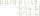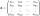Inverse matrix

Find how many times is the larger determinant is the matrix A, which equals 9 as the determinant of its inverse matrix.

Result

n =  81

Solution:Leave us a comment of example and its solution (i.e. if it is still somewhat unclear...):Be the first to comment!Next similar examples:

1. Inverse matrixFind out inverse by Gauss elimination or by reduction method. A=[2/3. 1 -3. 1/3]
2. The inverseThe inverse matrix for matrix A has a determinant value of 0.333. What value has a determinant of the matrix A?
3. The determinantThe determinant of the unit matrix equals 7. Check how many rows the A matrix contains.